# RS Aggarwal Solutions for Class 12 Maths Chapter 11: Applications of Derivatives Exercise 11C

Exercise 11C covers important concepts like Rolle’s and Lagrange’s Theorem along with its verification. Students can use the solutions designed by subject matter experts in order to boost their exam preparation. This mainly improves the problem solving abilities among students, which are important from the exam point of view. Students can obtain RS Aggarwal Solutions Class 12 Maths Chapter 11 Applications of Derivatives Exercise 11C PDF from the links which are given here.

## RS Aggarwal Solutions for Class 12 Maths Chapter 11: Applications of Derivatives Exercise 11C Download PDF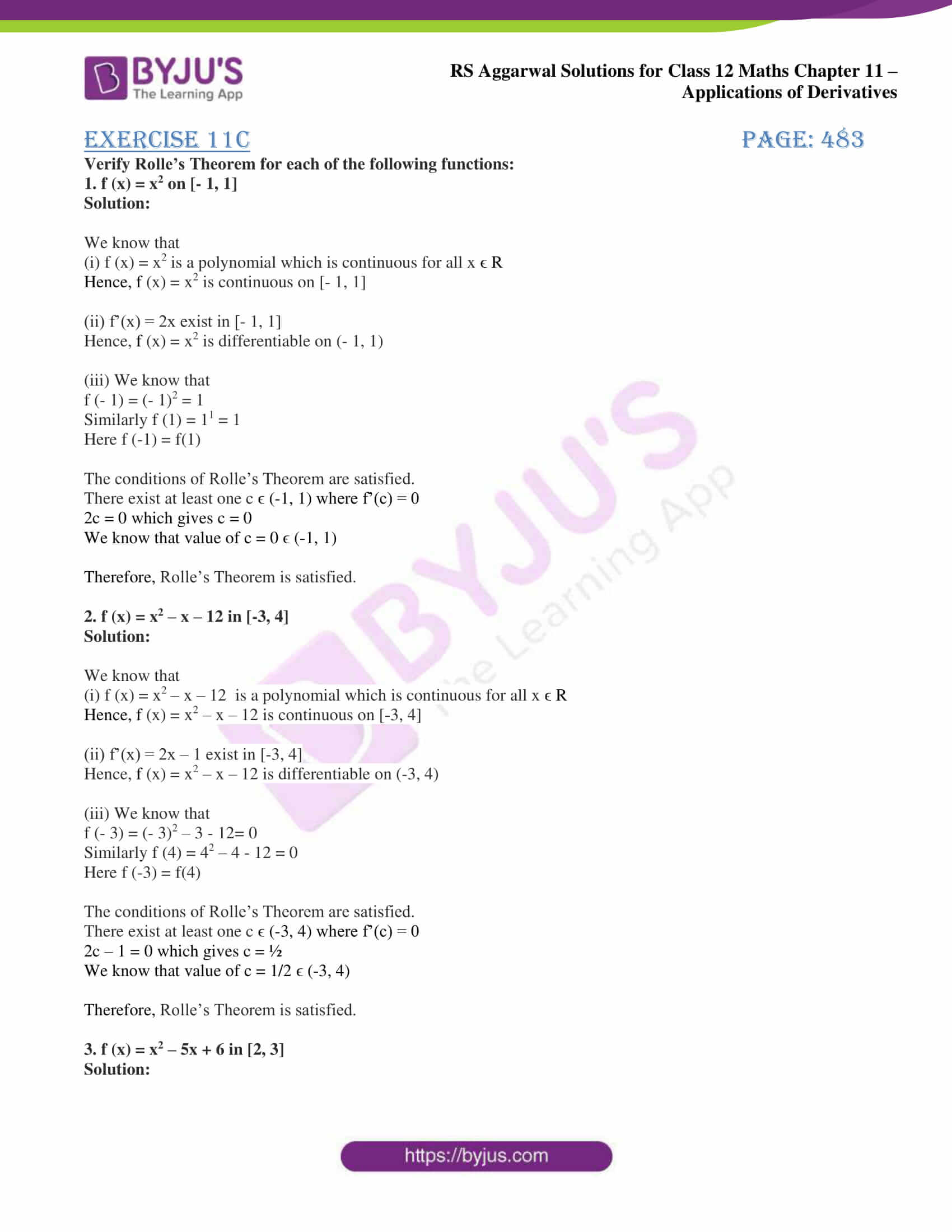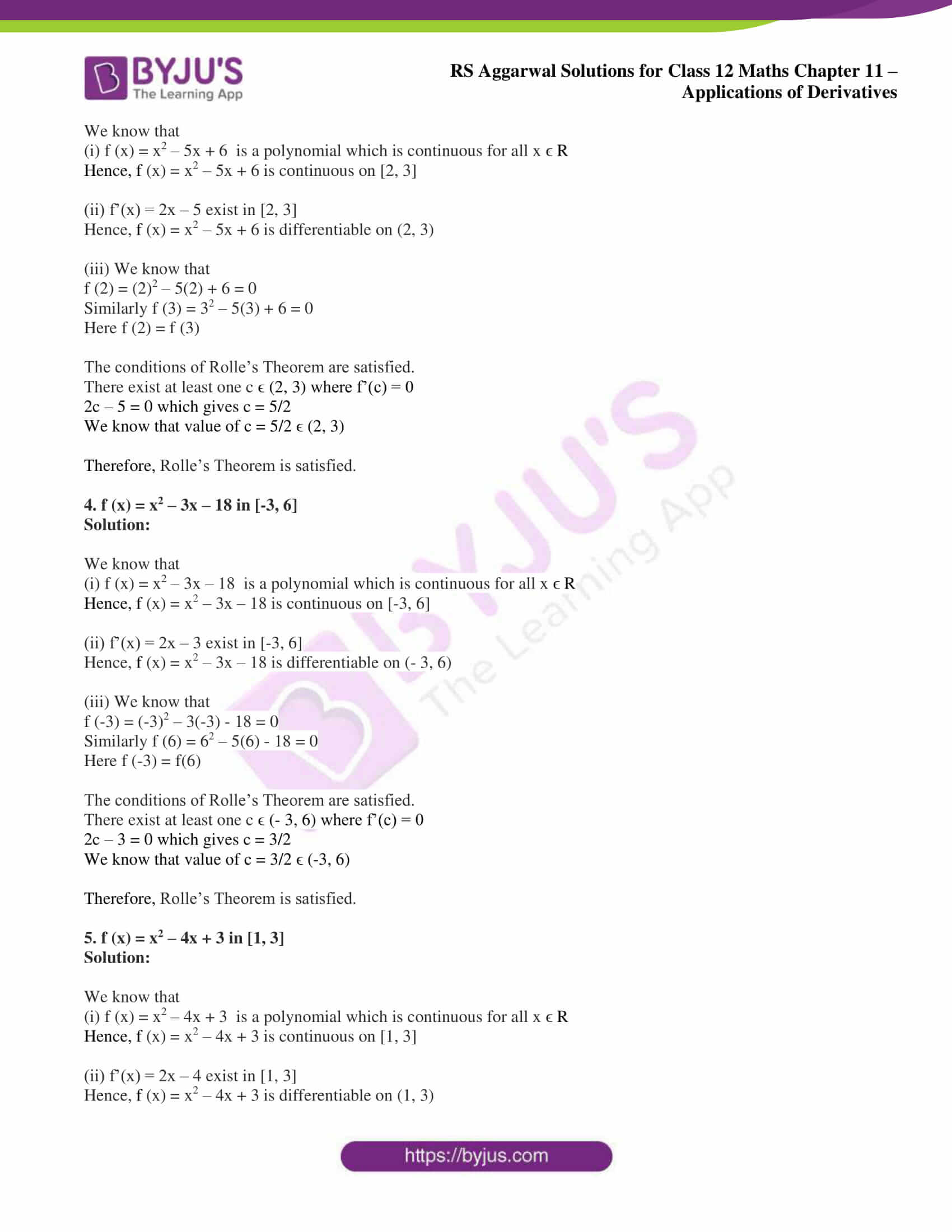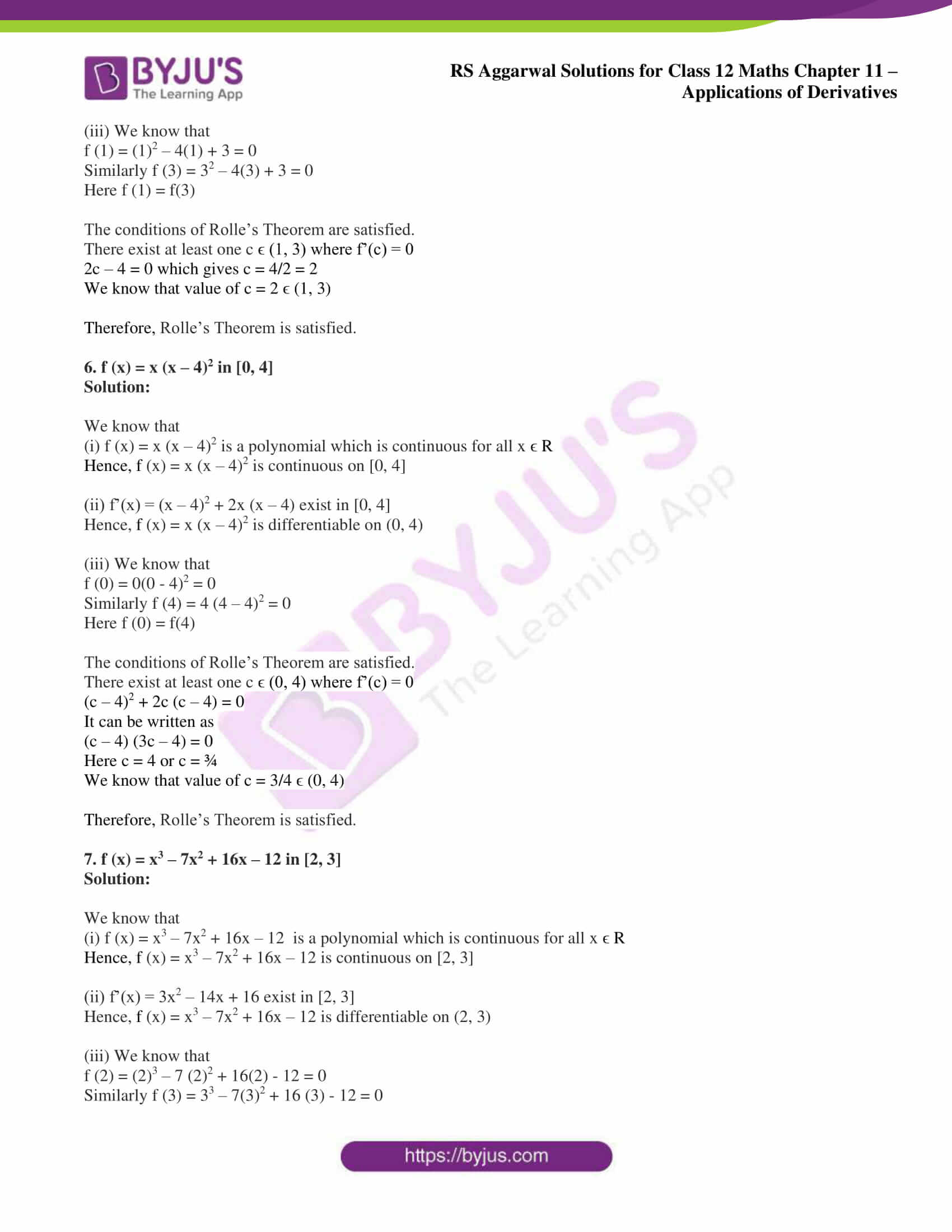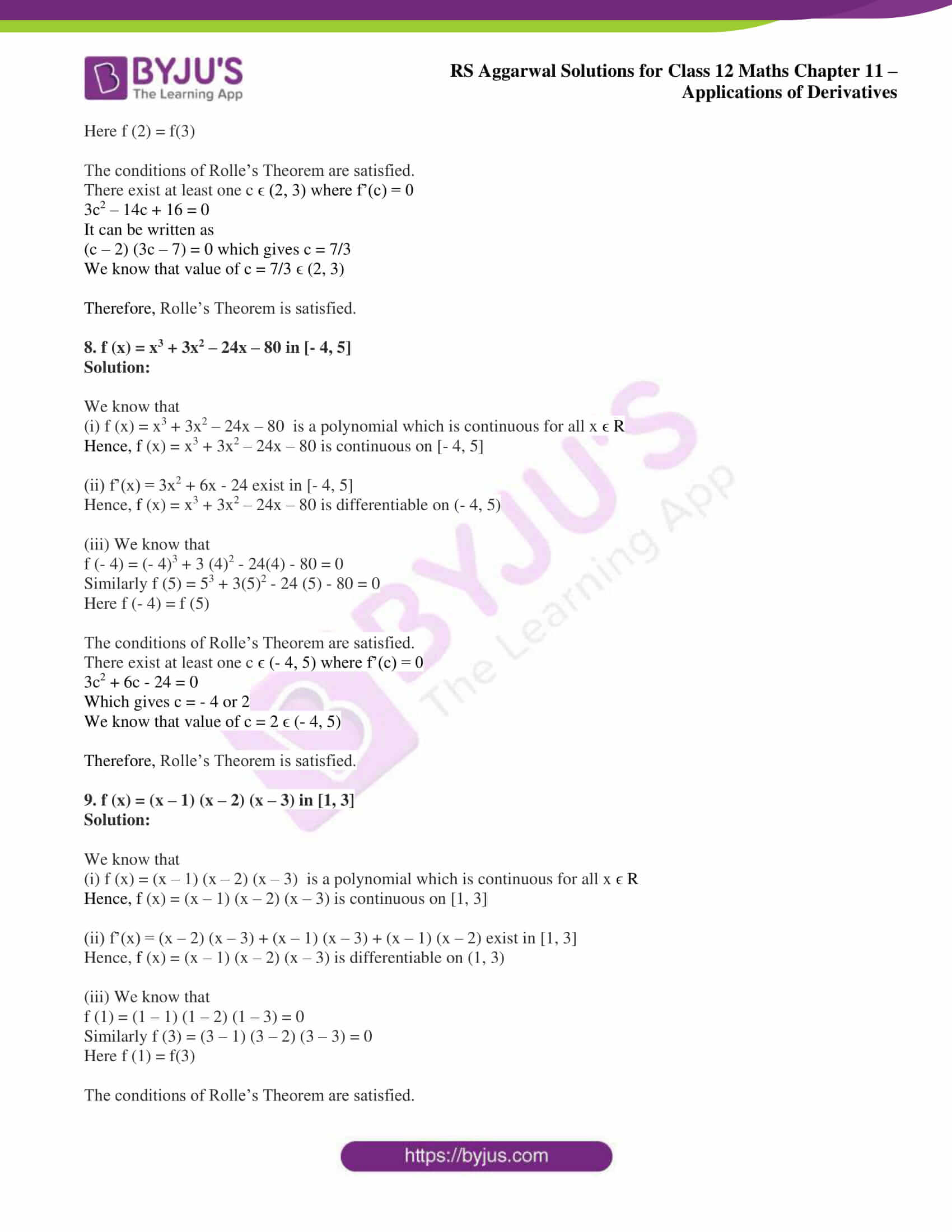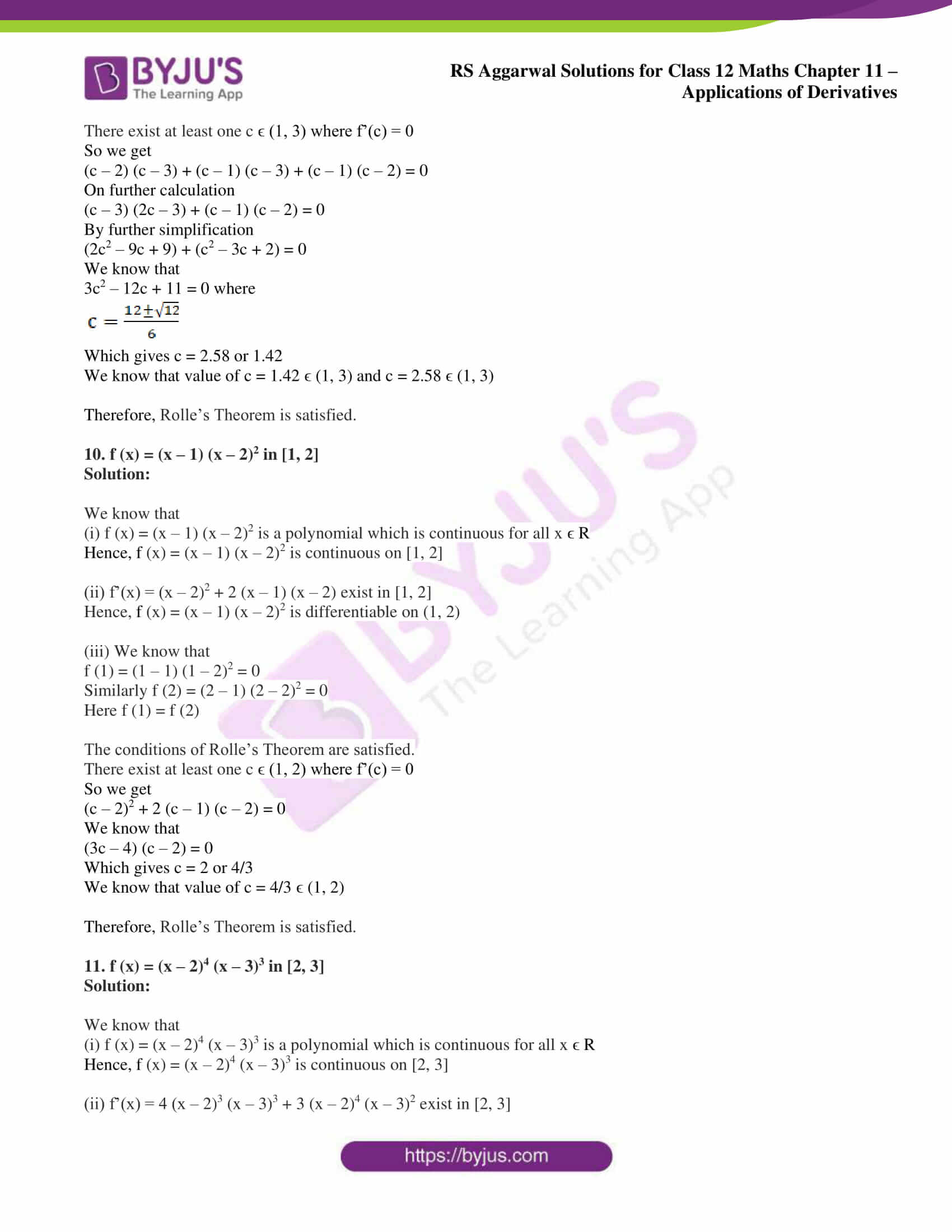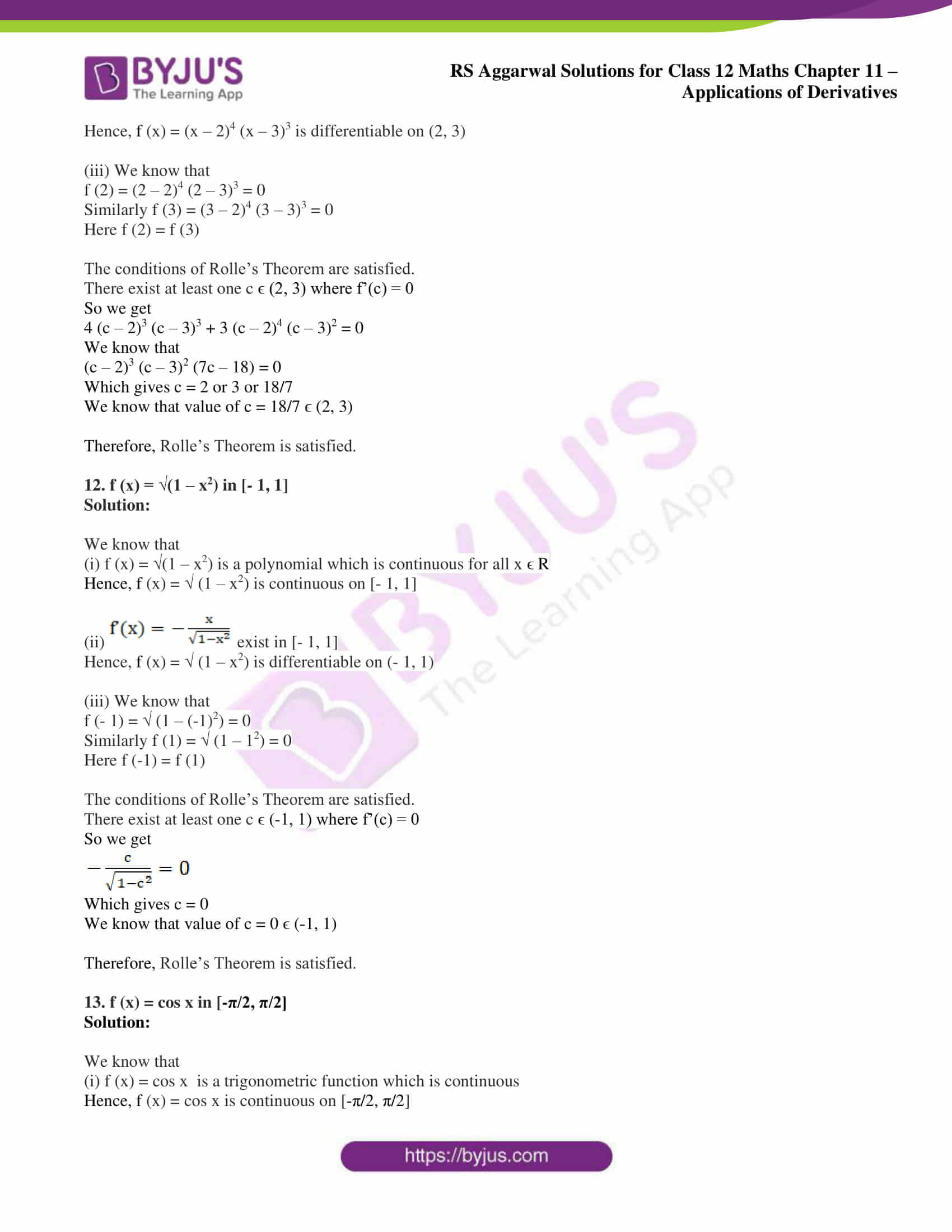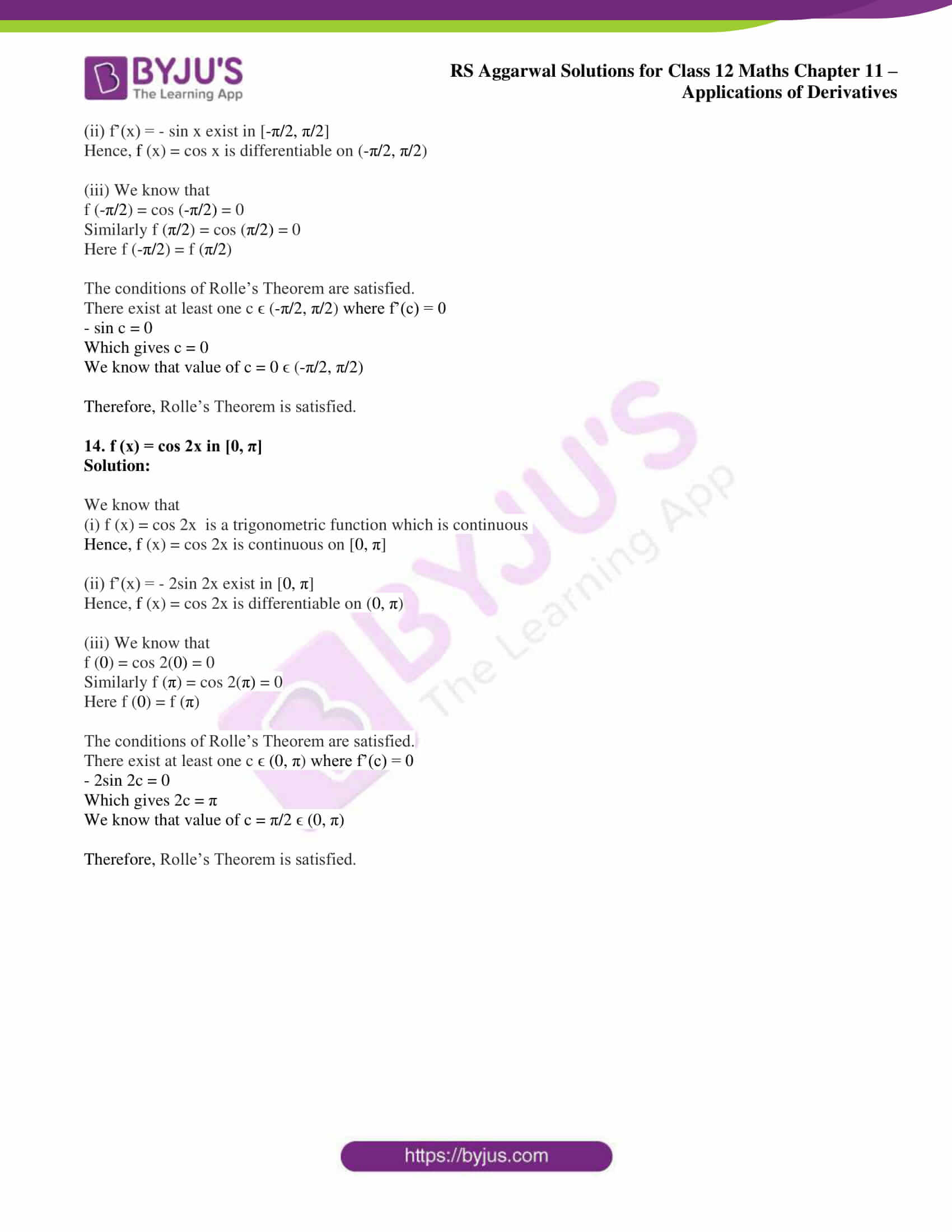### Access other exercise solutions of Class 12 Maths Chapter 11: Applications of Derivatives

Exercise 11A Solutions

Exercise 11B Solutions

Exercise 11D Solutions

Exercise 11E Solutions

Exercise 11F Solutions

Exercise 11G Solutions

Exercise 11H Solutions

### Access RS Aggarwal Solutions for Class 12 Maths Chapter 11: Applications of Derivatives Exercise 11C

Verify Rolle’s Theorem for each of the following functions:

1. f (x) = x2 on [- 1, 1]

Solution:

We know that

(i) f (x) = x2 is a polynomial which is continuous for all x ϵ R

Hence, f (x) = x2 is continuous on [- 1, 1]

(ii) f’(x) = 2x exist in [- 1, 1]

Hence, f (x) = x2 is differentiable on (- 1, 1)

(iii) We know that

f (- 1) = (- 1)2 = 1

Similarly f (1) = 11 = 1

Here f (-1) = f(1)

The conditions of Rolle’s Theorem are satisfied.

There exist at least one c ϵ (-1, 1) where f’(c) = 0

2c = 0 which gives c = 0

We know that value of c = 0 ϵ (-1, 1)

Therefore, Rolle’s Theorem is satisfied.

2. f (x) = x2 – x – 12 in [-3, 4]

Solution:

We know that

(i) f (x) = x2 – x – 12 is a polynomial which is continuous for all x ϵ R

Hence, f (x) = x2 – x – 12 is continuous on [-3, 4]

(ii) f’(x) = 2x – 1 exist in [-3, 4]

Hence, f (x) = x2 – x – 12 is differentiable on (-3, 4)

(iii) We know that

f (- 3) = (- 3)2 – 3 – 12= 0

Similarly f (4) = 42 – 4 – 12 = 0

Here f (-3) = f(4)

The conditions of Rolle’s Theorem are satisfied.

There exist at least one c ϵ (-3, 4) where f’(c) = 0

2c – 1 = 0 which gives c = ½

We know that value of c = 1/2 ϵ (-3, 4)

Therefore, Rolle’s Theorem is satisfied.

3. f (x) = x2 – 5x + 6 in [2, 3]

Solution:

We know that

(i) f (x) = x2 – 5x + 6 is a polynomial which is continuous for all x ϵ R

Hence, f (x) = x2 – 5x + 6 is continuous on [2, 3]

(ii) f’(x) = 2x – 5 exist in [2, 3]

Hence, f (x) = x2 – 5x + 6 is differentiable on (2, 3)

(iii) We know that

f (2) = (2)2 – 5(2) + 6 = 0

Similarly f (3) = 32 – 5(3) + 6 = 0

Here f (2) = f (3)

The conditions of Rolle’s Theorem are satisfied.

There exist at least one c ϵ (2, 3) where f’(c) = 0

2c – 5 = 0 which gives c = 5/2

We know that value of c = 5/2 ϵ (2, 3)

Therefore, Rolle’s Theorem is satisfied.

4. f (x) = x2 – 3x – 18 in [-3, 6]

Solution:

We know that

(i) f (x) = x2 – 3x – 18 is a polynomial which is continuous for all x ϵ R

Hence, f (x) = x2 – 3x – 18 is continuous on [-3, 6]

(ii) f’(x) = 2x – 3 exist in [-3, 6]

Hence, f (x) = x2 – 3x – 18 is differentiable on (- 3, 6)

(iii) We know that

f (-3) = (-3)2 – 3(-3) – 18 = 0

Similarly f (6) = 62 – 5(6) – 18 = 0

Here f (-3) = f(6)

The conditions of Rolle’s Theorem are satisfied.

There exist at least one c ϵ (- 3, 6) where f’(c) = 0

2c – 3 = 0 which gives c = 3/2

We know that value of c = 3/2 ϵ (-3, 6)

Therefore, Rolle’s Theorem is satisfied.

5. f (x) = x2 – 4x + 3 in [1, 3]

Solution:

We know that

(i) f (x) = x2 – 4x + 3 is a polynomial which is continuous for all x ϵ R

Hence, f (x) = x2 – 4x + 3 is continuous on [1, 3]

(ii) f’(x) = 2x – 4 exist in [1, 3]

Hence, f (x) = x2 – 4x + 3 is differentiable on (1, 3)

(iii) We know that

f (1) = (1)2 – 4(1) + 3 = 0

Similarly f (3) = 32 – 4(3) + 3 = 0

Here f (1) = f(3)

The conditions of Rolle’s Theorem are satisfied.

There exist at least one c ϵ (1, 3) where f’(c) = 0

2c – 4 = 0 which gives c = 4/2 = 2

We know that value of c = 2 ϵ (1, 3)

Therefore, Rolle’s Theorem is satisfied.

6. f (x) = x (x – 4)2 in [0, 4]

Solution:

We know that

(i) f (x) = x (x – 4)2 is a polynomial which is continuous for all x ϵ R

Hence, f (x) = x (x – 4)2 is continuous on [0, 4]

(ii) f’(x) = (x – 4)2 + 2x (x – 4) exist in [0, 4]

Hence, f (x) = x (x – 4)2 is differentiable on (0, 4)

(iii) We know that

f (0) = 0(0 – 4)2 = 0

Similarly f (4) = 4 (4 – 4)2 = 0

Here f (0) = f(4)

The conditions of Rolle’s Theorem are satisfied.

There exist at least one c ϵ (0, 4) where f’(c) = 0

(c – 4)2 + 2c (c – 4) = 0

It can be written as

(c – 4) (3c – 4) = 0

Here c = 4 or c = ¾

We know that value of c = 3/4 ϵ (0, 4)

Therefore, Rolle’s Theorem is satisfied.

7. f (x) = x3 – 7x2 + 16x – 12 in [2, 3]

Solution:

We know that

(i) f (x) = x3 – 7x2 + 16x – 12 is a polynomial which is continuous for all x ϵ R

Hence, f (x) = x3 – 7x2 + 16x – 12 is continuous on [2, 3]

(ii) f’(x) = 3x2 – 14x + 16 exist in [2, 3]

Hence, f (x) = x3 – 7x2 + 16x – 12 is differentiable on (2, 3)

(iii) We know that

f (2) = (2)3 – 7 (2)2 + 16(2) – 12 = 0

Similarly f (3) = 33 – 7(3)2 + 16 (3) – 12 = 0

Here f (2) = f(3)

The conditions of Rolle’s Theorem are satisfied.

There exist at least one c ϵ (2, 3) where f’(c) = 0

3c2 – 14c + 16 = 0

It can be written as

(c – 2) (3c – 7) = 0 which gives c = 7/3

We know that value of c = 7/3 ϵ (2, 3)

Therefore, Rolle’s Theorem is satisfied.

8. f (x) = x3 + 3x2 – 24x – 80 in [- 4, 5]

Solution:

We know that

(i) f (x) = x3 + 3x2 – 24x – 80 is a polynomial which is continuous for all x ϵ R

Hence, f (x) = x3 + 3x2 – 24x – 80 is continuous on [- 4, 5]

(ii) f’(x) = 3x2 + 6x – 24 exist in [- 4, 5]

Hence, f (x) = x3 + 3x2 – 24x – 80 is differentiable on (- 4, 5)

(iii) We know that

f (- 4) = (- 4)3 + 3 (4)2 – 24(4) – 80 = 0

Similarly f (5) = 53 + 3(5)2 – 24 (5) – 80 = 0

Here f (- 4) = f (5)

The conditions of Rolle’s Theorem are satisfied.

There exist at least one c ϵ (- 4, 5) where f’(c) = 0

3c2 + 6c – 24 = 0

Which gives c = – 4 or 2

We know that value of c = 2 ϵ (- 4, 5)

Therefore, Rolle’s Theorem is satisfied.

9. f (x) = (x – 1) (x – 2) (x – 3) in [1, 3]

Solution:

We know that

(i) f (x) = (x – 1) (x – 2) (x – 3) is a polynomial which is continuous for all x ϵ R

Hence, f (x) = (x – 1) (x – 2) (x – 3) is continuous on [1, 3]

(ii) f’(x) = (x – 2) (x – 3) + (x – 1) (x – 3) + (x – 1) (x – 2) exist in [1, 3]

Hence, f (x) = (x – 1) (x – 2) (x – 3) is differentiable on (1, 3)

(iii) We know that

f (1) = (1 – 1) (1 – 2) (1 – 3) = 0

Similarly f (3) = (3 – 1) (3 – 2) (3 – 3) = 0

Here f (1) = f(3)

The conditions of Rolle’s Theorem are satisfied.

There exist at least one c ϵ (1, 3) where f’(c) = 0

So we get

(c – 2) (c – 3) + (c – 1) (c – 3) + (c – 1) (c – 2) = 0

On further calculation

(c – 3) (2c – 3) + (c – 1) (c – 2) = 0

By further simplification

(2c2 – 9c + 9) + (c2 – 3c + 2) = 0

We know that

3c2 – 12c + 11 = 0 where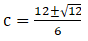Which gives c = 2.58 or 1.42

We know that value of c = 1.42 ϵ (1, 3) and c = 2.58 ϵ (1, 3)

Therefore, Rolle’s Theorem is satisfied.

10. f (x) = (x – 1) (x – 2)2 in [1, 2]

Solution:

We know that

(i) f (x) = (x – 1) (x – 2)2 is a polynomial which is continuous for all x ϵ R

Hence, f (x) = (x – 1) (x – 2)2 is continuous on [1, 2]

(ii) f’(x) = (x – 2)2 + 2 (x – 1) (x – 2) exist in [1, 2]

Hence, f (x) = (x – 1) (x – 2)2 is differentiable on (1, 2)

(iii) We know that

f (1) = (1 – 1) (1 – 2)2 = 0

Similarly f (2) = (2 – 1) (2 – 2)2 = 0

Here f (1) = f (2)

The conditions of Rolle’s Theorem are satisfied.

There exist at least one c ϵ (1, 2) where f’(c) = 0

So we get

(c – 2)2 + 2 (c – 1) (c – 2) = 0

We know that

(3c – 4) (c – 2) = 0

Which gives c = 2 or 4/3

We know that value of c = 4/3 ϵ (1, 2)

Therefore, Rolle’s Theorem is satisfied.

11. f (x) = (x – 2)4 (x – 3)3 in [2, 3]

Solution:

We know that

(i) f (x) = (x – 2)4 (x – 3)3 is a polynomial which is continuous for all x ϵ R

Hence, f (x) = (x – 2)4 (x – 3)3 is continuous on [2, 3]

(ii) f’(x) = 4 (x – 2)3 (x – 3)3 + 3 (x – 2)4 (x – 3)2 exist in [2, 3]

Hence, f (x) = (x – 2)4 (x – 3)3 is differentiable on (2, 3)

(iii) We know that

f (2) = (2 – 2)4 (2 – 3)3 = 0

Similarly f (3) = (3 – 2)4 (3 – 3)3 = 0

Here f (2) = f (3)

The conditions of Rolle’s Theorem are satisfied.

There exist at least one c ϵ (2, 3) where f’(c) = 0

So we get

4 (c – 2)3 (c – 3)3 + 3 (c – 2)4 (c – 3)2 = 0

We know that

(c – 2)3 (c – 3)2 (7c – 18) = 0

Which gives c = 2 or 3 or 18/7

We know that value of c = 18/7 ϵ (2, 3)

Therefore, Rolle’s Theorem is satisfied.

12. f (x) = √(1 – x2) in [- 1, 1]

Solution:

We know that

(i) f (x) = √(1 – x2) is a polynomial which is continuous for all x ϵ R

Hence, f (x) = √ (1 – x2) is continuous on [- 1, 1]

(ii)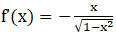exist in [- 1, 1]

Hence, f (x) = √ (1 – x2) is differentiable on (- 1, 1)

(iii) We know that

f (- 1) = √ (1 – (-1)2) = 0

Similarly f (1) = √ (1 – 12) = 0

Here f (-1) = f (1)

The conditions of Rolle’s Theorem are satisfied.

There exist at least one c ϵ (-1, 1) where f’(c) = 0

So we getWhich gives c = 0

We know that value of c = 0 ϵ (-1, 1)

Therefore, Rolle’s Theorem is satisfied.

13. f (x) = cos x in [-π/2, π/2]

Solution:

We know that

(i) f (x) = cos x is a trigonometric function which is continuous

Hence, f (x) = cos x is continuous on [-π/2, π/2]

(ii) f’(x) = – sin x exist in [-π/2, π/2]

Hence, f (x) = cos x is differentiable on (-π/2, π/2)

(iii) We know that

f (-π/2) = cos (-π/2) = 0

Similarly f (π/2) = cos (π/2) = 0

Here f (-π/2) = f (π/2)

The conditions of Rolle’s Theorem are satisfied.

There exist at least one c ϵ (-π/2, π/2) where f’(c) = 0

– sin c = 0

Which gives c = 0

We know that value of c = 0 ϵ (-π/2, π/2)

Therefore, Rolle’s Theorem is satisfied.

14. f (x) = cos 2x in [0, π]

Solution:

We know that

(i) f (x) = cos 2x is a trigonometric function which is continuous

Hence, f (x) = cos 2x is continuous on [0, π]

(ii) f’(x) = – 2sin 2x exist in [0, π]

Hence, f (x) = cos 2x is differentiable on (0, π)

(iii) We know that

f (0) = cos 2(0) = 0

Similarly f (π) = cos 2(π) = 0

Here f (0) = f (π)

The conditions of Rolle’s Theorem are satisfied.

There exist at least one c ϵ (0, π) where f’(c) = 0

– 2sin 2c = 0

Which gives 2c = π

We know that value of c = π/2 ϵ (0, π)

Therefore, Rolle’s Theorem is satisfied.